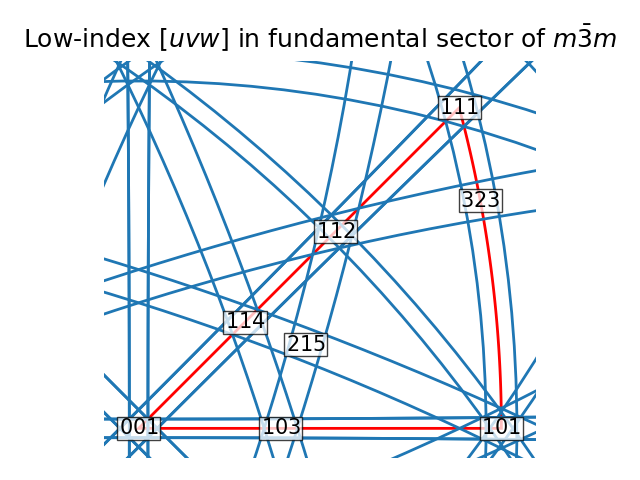# Restrict to fundamental sector#

This example shows how to restrict the stereographic plot to only the fundamental sector of a point group using restrict_to_sector(). The sector is typically obtained from orix.quaternion.Symmetry.fundamental_sector, and is often called the ‘fundamental triangle’.

We demonstrate this functionality by drawing (near) great circles about some typically strongly reflecting low-index reciprocal lattice vectors $$\{hkl\}$$ in crystals of point group $$m\bar{3}m$$. The deviations from the great circles are related to the kinematically calculated width of a Kikuchi band scattered from these vectors assuming a lattice parameter of 4.04 Å (Aluminium) and an accelerating voltage of 20 keV.Out:

Miller (4,), point group m-3m, hkl
[[1. 1. 1.]
[2. 0. 0.]
[2. 2. 0.]
[3. 1. 1.]]


import numpy as np

from orix.crystal_map import Phase
from orix.quaternion import symmetry
from orix.vector import Miller

# Symmetrically equivalent set of hkl
hkl1 = Miller(
hkl=[[1, 1, 1], [2, 0, 0], [2, 2, 0], [3, 1, 1]],
phase=Phase(point_group=symmetry.Oh),
)
print(hkl1)
hkl, idx = hkl1.symmetrise(unique=True, return_index=True)

# Width of Kikuchi bands (deviation from great circles)
theta = np.deg2rad([1.054, 1.218, 1.722, 2.019])
theta = theta[idx]

# Plot pair of near great circles
fig = hkl.draw_circle(opening_angle=np.pi / 2 + theta, return_figure=True)
hkl.draw_circle(opening_angle=np.pi / 2 - theta, figure=fig)

# Restrict to fundamental sector of m-3m (with some padding outside sector)
ax = fig.axes
ax.restrict_to_sector(
)

# Get symmetrically equivalent set of zone axes <uvw>
uvw = hkl.reshape(hkl.size, 1).cross(hkl.reshape(1, hkl.size)).flatten()
uvw = uvw.in_fundamental_sector()
uvw = uvw.unique(use_symmetry=True)
uvw = uvw.round()

for uvw_i in uvw:
uvw_idx = str(uvw_i.coordinates.astype(int)).replace(" ", "")
ax.text(
uvw_i,
s=uvw_idx,
va="bottom",
_ = ax.set_title(r"Low-index $[uvw]$ in fundamental sector of $m\bar{3}m$", pad=10)